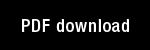Fuzzy ARTMAP, Slow Learning, and Probability Estimation

Author(s): Carpenter, G.A. | Grossberg, S. | Reynolds, J.H. |

Year: 1993

Citation: Proceedings of the World Congress on Neural Networks (WCNN 93), II 26-30.

Abstract: A nonparametric probability estimation procedure using the fuzzy ARTMAP neural network is here described. Because the procedure does not make a priori assumptions about underlying probability distributions, it yields accurate estimates on a wide variety of prediction tasks. Fuzzy ARTMAP is used to perform probability estimation in two different modes. In a slow learning mode, input-output associations change slowly, with the strength of each association computing a conditional probability estimate. In max-nodes mode, a fixed number of categories are coded during an initial fast learning interval, and weights are then tuned by slow learning. Simulations illustrate system performance on tasks in which various numbers of clusters in the set of input vectors mapped to a given class.

Topics: Machine Learning, Models: Fuzzy ARTMAP,